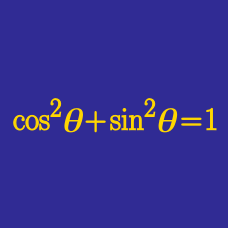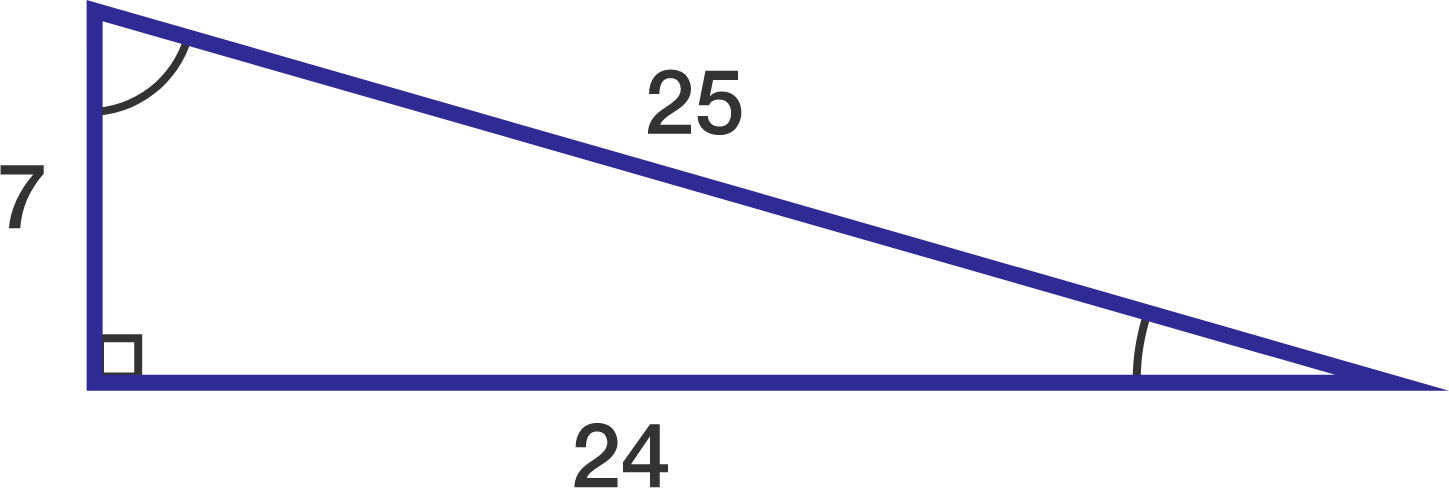Geometry

# Fundamental Trigonometric Identities: Level 4 Challenges

Evaluate the sum

\begin{aligned} & \ \ \ \log_{\cos1}(\tan{1}) \\ &+\log_{\cos{2}}(\tan{2}) \\ &+ \log_{\cos{3}}(\tan{3}) \\& +\ldots \\ &+ \log_{\cos{44}} (\tan{44}) \\ &+ \log_{\sin{45}}(\tan{45}) \\ &+ \log_{\sin46}(\tan{46}) \\ &+\ldots \\ &+ \log_{\sin89}(\tan{89}). \end{aligned}

Note: All angles are in degrees, and be aware that the base changes from $\cos$ to $\sin$.

$\large (7\cos x+24\sin x)(7\sin x-24\cos x)$

Find the maximum value of this expression over all real values $x.$

Hint:If $x$ and $y$ are acute angles such that

$\frac {\sin x}{\sin y } = \frac {1}{2}, \quad \frac {\cos x}{\cos y } = \frac 3 2 ,$

what is $\tan^2 (x+y)?$

Define the function $f(x)=\frac{2x}{1-x^2}$. Find the number of distinct real solutions of the equation $f^{(5)} (x) =x.$

Details and assumptions

$f^{(n)} (x)$ denotes the function $f$ applied $n$ times. In particular, $f^{(5)} (x) = f(f(f(f(f(x)))))$.

Find $\cos{1˚} \cos{2˚}+\cos{2˚} \cos{3˚}+ \cdots +\cos{88˚} \cos{89˚}.$